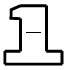Home > CC1MN > Chapter cc13 > Lesson cc13.1.1 > Problem3-12

3-12.

Copy and complete each of the following Giant One problems. Homework Help ✎

1. $\frac{5}{3}\cdot$$=\ \frac{ }{18}$

• By what number do you multiply 3 to get 18? This will be both the numerator and denominator in the Giant One, since the fraction is equal to one.

$\left(\frac{5}{3}\right) \! \left(\frac{6}{6}\right) \! =\frac{30}{18}$

1. $\frac{28}{63}\cdot$$=\ \frac{4}{ }$

• Follow the same method as in part (a). What number times 4 is equal to 28?

1. $\frac{9}{20}\cdot$$=\ \frac{ }{100}$

• This problem is very similar to part (a). Remember that the Giant One is a fraction equal to one.